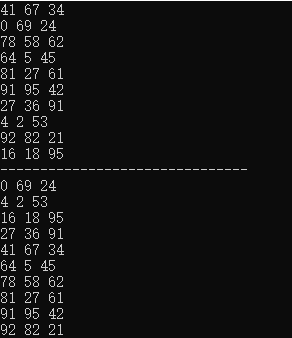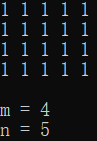• 1.sort()函数，默认的是对二维数组按照第一列的大小对每行的数组进行排序。默认从小到大 #include <iostream> #include<vector> #include<algorithm> using namespace std; int main() { vector...
1.sort()函数，默认的是对二维数组按照第一列的大小对每行的数组进行排序。默认从小到大
#include <iostream>
#include<vector>
#include<algorithm>
using namespace std;

int main()
{
vector<vector<int> > intervals(10);
for (int i = 0; i < 10; i++)
{
for (int j = 0; j < 3; j++)
{
intervals[i].push_back(rand() % 100);
}
}
for (int i = 0; i < 10; i++)
{
for (int j = 0; j < 3; j++)
{
cout<<intervals[i][j]<<" ";
}
cout<<endl;
}
sort(intervals.begin(),intervals.end());
cout<<"-------------------------------"<<endl;
for (int i = 0; i < 10; i++)
{
for (int j = 0; j < 3; j++)
{
cout<<intervals[i][j]<<" ";
}
cout<<endl;
}
return 0;
}


结果：自定义比较函数：按照第一列从大到小，如果相等则按照第二列从小到大
bool cmp(vector<int>&a,vector<int>&b){
if(a!=b) return a>b;
else return a<b;
}
sort(intervals.begin(),intervals.end(),cmp);

完整代码：
#include <iostream>
#include<vector>
#include<algorithm>
using namespace std;
bool cmp(vector<int>&a,vector<int>&b){
if(a!=b) return a>b;
else return a<b;
}
int main()
{
vector<vector<int> > intervals(10);
for (int i = 0; i < 10; i++)
{
for (int j = 0; j < 3; j++)
{
intervals[i].push_back(rand() % 100);
}
}
for (int i = 0; i < 10; i++)
{
for (int j = 0; j < 3; j++)
{
cout<<intervals[i][j]<<" ";
}
cout<<endl;
}
sort(intervals.begin(),intervals.end(),cmp);
cout<<"-------------------------------"<<endl;
for (int i = 0; i < 10; i++)
{
for (int j = 0; j < 3; j++)
{
cout<<intervals[i][j]<<" ";
}
cout<<endl;
}
return 0;
}


或者写作：
sort(intervals.begin(),intervals.end(),[](const vector<int>& a,const vector<int>& b){
return  a<b;
});

展开全文• C++获取vector二维数组的行数和数 代码： #include <iostream> #include <string> using namespace std; int main(){ //创建4*5二维数组，并将数组元素的值都设为1 vector<vector<int>>...
C++获取vector二维数组的行数和列数 代码：
#include <iostream>
#include <string>
#include <vector>
using namespace std;

int main(){
//创建4*5二维数组，并将数组元素的值都设为1
vector<vector<int>> dp(4, vector<int>(5, 1));
//打印二维数组
for (int i = 0; i < 4; i++) {
for (int j = 0; j < 5; j++)
cout << dp[i][j] << " ";
cout << endl;
}
cout << endl;

int m = dp.size();//行数
int n = dp.size();//列数
cout << "m = " << m << endl;
cout << "n = " << n << endl;
return 0;
}

结果：展开全文c++
• //与sort一起写在同个函数里面 void do_sth(vector<vector<int>>&vec)//有些编译器>>之间要有空格 //&可避免直接复制数组，提高编译效率 auto cmp = [](vector<int>& a, ...
与sort一起写在同一个函数里面
void do_sth(vector<vector<int>>&vec)//有些编译器>>之间要有空格
//&可避免直接复制数组，提高编译效率
{
auto cmp = [](vector<int>& a, vector<int>&b）
{return a<b;//表示按第0列从小到大进行排序};
sort(vec.begin(),vec.end(),cmp);
}

编写一个compare函数
bool compare(vector<int>&a,vector<int>&b)
{return a<b;}
void do_sth(vector<vector<int>>&vec)
{
...
sort(vec.begin(),vec.end(),compare);
...
}

如果没通过可将函数头改成 static bool compare()
还有一种方法是将vector<vector<int>>vec替换成vector<pair<int,int>>vec，不过我暂时还没搞懂具体操作QAQ
展开全文• ## vector二维数组

千次阅读 2020-10-20 21:56:22
//注意，int[m]表示个有m个元素的指针数组 //p=new int(8)表示分配个int空间并初始化为8； for (int i = 0; i < m; ++i) { p[i] = new int; } 利用Vector的push_back函数 vector<vector<int...
动态数组
int *p;
p = new int[m];    //注意，int[m]表示一个有m个元素的指针数组
//p=new int(8)表示分配一个int空间并初始化为8；

for (int i = 0; i < m; ++i)
{
p[i] = new int;
}利用Vector的push_back函数
vector<vector<int>> vec; //外层
vector<int> a; 			//内层
a.push_back(1);
a.push_back(2);
a.push_back(3);

vector<int> b;
b.push_back(4);
b.push_back(5);
b.push_back(6);

vec.push_back(a);
vec.push_back(b);

先定义好二维数组结构，在直接赋值
vector<vector<int> > array(m); //m表示的是初始化大小=array.size()，而不是初始值
//初始化一个m*n的二维数组
for(int i=0;i<m;i++)
{
array[i].resize(n);
}
//遍历赋值
for(i = 0; i < array.size(); i++)
{
for (j = 0; j < array.size();j++)
{
array[i][j] = (i+1)*(j+1);
}
}

遍历
（1）利用迭代器
void reverse_with_iterator(vector<vector<int>> vec)
{
if (vec.empty())
{
cout << "The vector is empty!" << endl;
return;
}

vector<int>::iterator it;
vector<vector<int>>::iterator iter;
vector<int> vec_tmp;

cout << "Use iterator : " << endl;
for(iter = vec.begin(); iter != vec.end(); iter++)
{
vec_tmp = *iter;
for(it = vec_tmp.begin(); it != vec_tmp.end(); it++)
cout << *it << " ";
cout << endl;
}
}

（2）得到行、列大小，利用下标进行遍历（推荐）
void reverse_with_index(vector<vector<int>> vec)
{
if (vec.empty())
{
cout << "The vector is empty!" << endl;
return;
}

int i,j;
cout << "Use index : " << endl;
for (i = 0; i < vec.size(); i++)
{
for(j = 0; j < vec.size(); j++)
cout << vec[i][j] << " ";
cout << endl;
}
}参考链接: link. 参考链接: link.
展开全文• C++ STL库中的vector类似于封装了个动态的数组，非常的好用，关于vector的一些函数，见这篇博客： ...首先是一维数组的创建，一维数组可以直接创建，也可以通过数组的方式创建 int arr[] = {1, 2,...
• 在完成CodeForce 234E的时候,想到可以用Vector来完成模拟,下面给出代码 #include<bits/stdc++.h> using namespace std; struct Team{ char name; int score; } team; bool cmp(Team A,Team B) { ...c++ 算法 stl
• 个bool类型的comp函数，比如下面根据第个元素排序： bool cmp1(const vector<int> &a, const vector<int> &b){ return a > b; } sort(allvec.begin(), allvec.end(), cmp1...
• 在一个二维数组中，每一行都按照从左到右递增的顺序排序，每一列都按照从上到下递增的顺序排序。请完成一个函数，输入这样的一个二维数组和一个整数，判断数组中是否含有该整数。 解题思路： 第一行到最后一行是...剑指offer
• 1. vector二维数组初始化方法: 2.示例代码 unsigned int rows = costMatrix.rows(); unsigned int cols = costMatrix.cols(); vector< vector<double> > costFunc(rows,vector<double>...
• 在一个 n * m的二维数组中，每一行都按照从左到右递增的顺序排序，每一列都按照从上到下递增的顺序排序。请完成一个高效的函数，输入这样的一个二维数组和一个整数，判断数组中是否含有该整数。 一、解题思路 从...
• 在刷LeetCode的时候需要初始化创建个行大小为r、大小为c的vector二维数组，具体的实现方法如下： vector<vector<int>> num(r, vector<int>(c)); 初始化创建r个类型为vector<int>，大小...c++ leetcode 开发语言
• vector二维数组注意使用前要分配空间，不然就会出现以下错误： error: reference binding to null pointer of type 'value_type' 错误不唯一 所以最好在vector二维数组定义时就分配好空间 vector<vector<int&...c++
• 之前我一直以为vector不能vec = {1,2,3}这种方式直接定义，直到我看到下面的代码 vector<vector<int> >num = { { 1, 1, 1, 0, 1, 1 }, { 1, 0, 1, 1, 1, 1 }, { 1, 1, 1, 0, 0, 1 }, { 1, 0, 1, 0,...
• vector<vector <int> > array(3);...array.size()//返回二维数组行的数 定义个行列固定的二维vector数组 例： vector<vector<int> > a; a.resize(m);//...
• 固定大小： #include <iostream> #include <vector> using namespace std; int main() { int row, column; cin >> row >... vector<vector<... a(row, vector<... //使用c++ 数据结构 经验分享
• ## vector二维数组使用

千次阅读 2017-08-31 15:46:32
本文综述了二维数组使用，代码可以跑一下看看效果。 #include #include using namespace std; //method 1 /* int main() { int row, column; cin >> row >> column; //申请空间 vector > a; a.resize(row, ...
• vector<vector<int> >num = { { 1，2，3，4 }, { 2，3，4，5}, { 4，5，6，7 }, { 6，7，8，9 } }; vector<vector<int> >nums(n,vector<int>(m,0)); //n行m算法
• ## Vector 实现二维数组

千次阅读 2020-11-24 21:01:25
1. 这里简单叙述一下C++ 构建二维动态数组 int **p; p = new int*; //注意，int*表示个有10个元素的指针数组 for (int i = 0; i < 10; ++i) { p[i] = new int; } 2. 初始化 方法1 vector<...c++
• vector二维数组的内存分析 创建个2x4的二维数组。想到的办法是：先创建个容器的容器，外层大小的2（2行），然后里面容器小大为4（4）。 int row=2,col=4; vector<vector<int> > v(row);//v为...
• #include <iostream> using namespace std; #include <vector> int main() ... int r = 0, c = 0;...//规定二维数组列数 cin >> c; vector<vector<int>> array;//定义二
• 二维数组排序数组排序有内置API，一般是一维的，这里分享下二维或者多维的用法1 C++ 二维数组一列升序，第一列相同第二列降序2 C 二维数组一列升序，第一列相同第二列降序3 更一般的思路 数组排序有内置API，...
• 自己本地调试代码的时候，老是需要用vector创建个已知行数，数的二维数组，所以，总结一下，vector数组的初始化问题。
• 在做项目时，需要对二维数组，按照指定的进行排序。 效果图： 代码实现： public static void main(String[] args) { int[][] nums = initialArray(5, 3); System.out.println("排序前："); printAr...排序 Java
• 用法： 在C++中，头文件加上#include，在函数中加上语句： ...// vi~vi每个都是vector容器 举个例子： 代码： #include <iostream> #include <vector> using namespace std; int...c++
• 一维数组定义方法： #include &lt;iostream&gt; #include &lt;vector&gt; using namespace std; int main() { vector&lt;int&gt; arr(10); //arr的长度为10 cout&lt;&lt;arr....
• ## vector定义二维数组

千次阅读 2019-09-05 21:49:36
problem: 给定n阶方阵里所有的数，求方阵里...方案使用vector #include <iostream> using namespace std; #include <vector> #include <algorithm> int main() { int n,num; int sum=0; ...leetcode
• 在完成项任务时可能会需要用到动态二维数组，在C++中，可以使用二维vector创建二维数组。对二维数组使用，其操作可分为增删查改。其中修改操作可以通过简单赋值操作完成，之后便不再赘述。 创建二维数组： ...二维数组 动态二维数组
• int main() { vector<vector<int>> dp(5, vector<int>(10,3)); //创建5*10二维数组 dp.push_back(vector<int>(10,5)); //添加行，成为了6*10数组 dp = 100; ...
• 维和二维动态数组初始化为： std::vector vec(10,90); //将10个维动态数组初始为90 std::vector > vec(row,vector(col,0)); //初始化row * col二维动态数组，初始化值为0C++
• ## C++ vector 实现二维数组

万次阅读 多人点赞 2016-08-01 20:25:04
在STL中Vector容器，无论是在封装程度还是内存管理等方面都由于传统C++中的数组。本文主要是关于使用Vector初始化、遍历方面的内容。其他二维的思想也是类似的。 1.初始化 （1）利用Vector的push_back函数...C++...